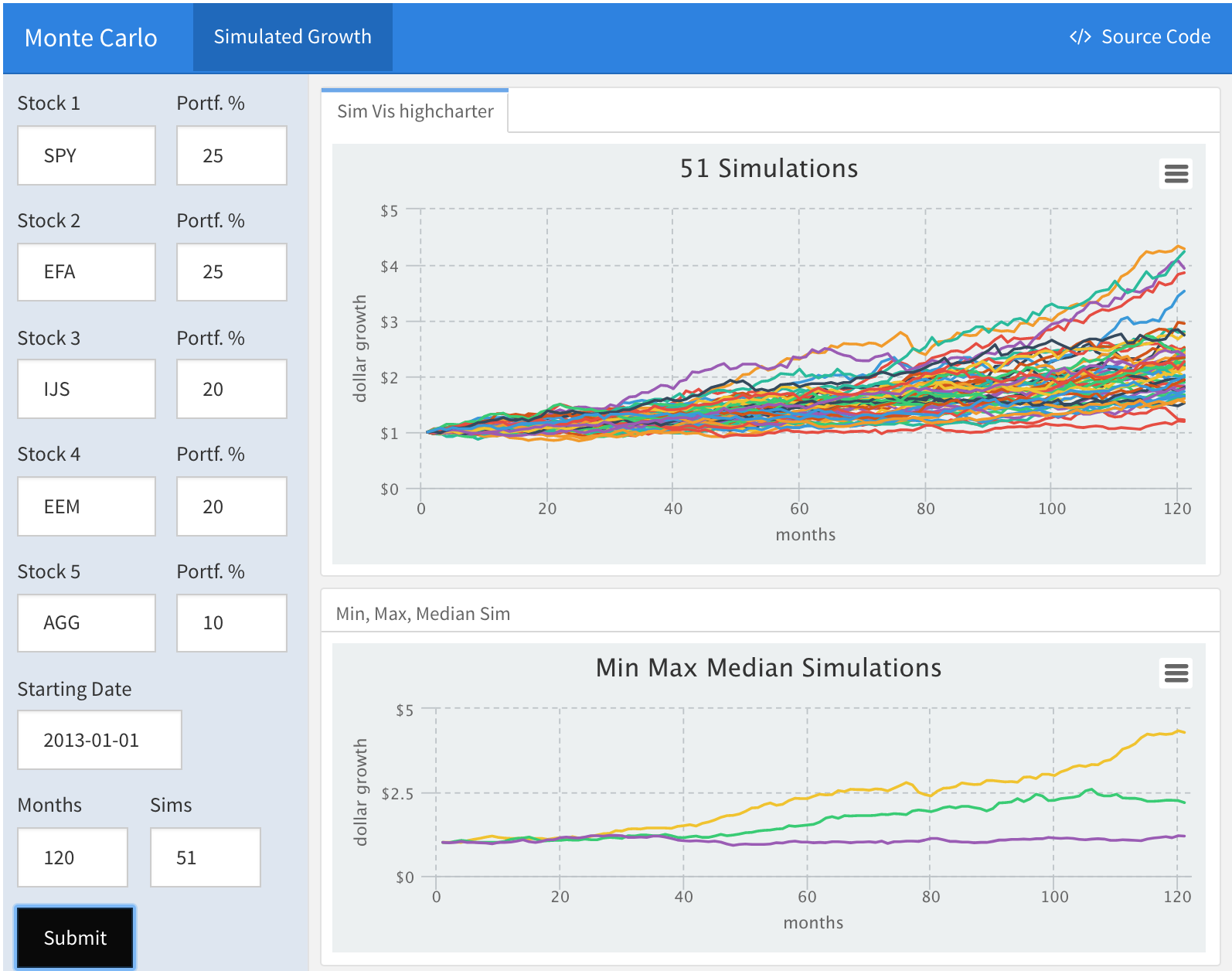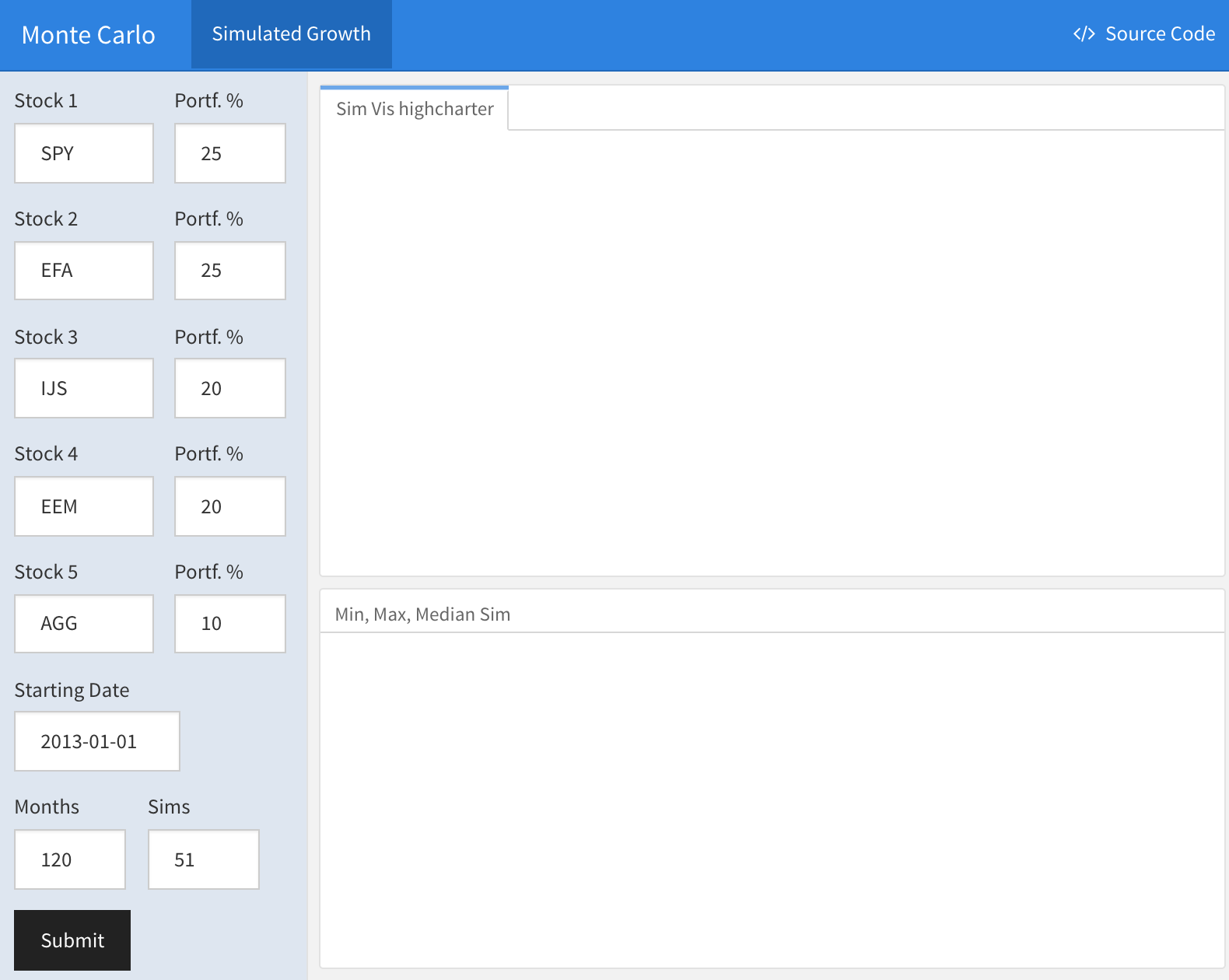# Monte Carlo Shiny: Part Three

by Jonathan Regenstein

In previous posts, we covered how to run a Monte Carlo simulation and how to visualize the results. Today, we will wrap that work into a Shiny app wherein a user can build a custom portfolio, and then choose a number of simulations to run and a number of months to simulate into the future.

A link to that final Shiny app is here and here is a snapshot:We will use RMarkdown to build our Shiny application by inserting runtime: shiny into the yaml header. This will alert the server (or our laptop) that this is an interactive document. The yaml header also gives us a space for the title and to specify the format as flexdashboard. This is what the yaml header looks like for the app.

---
title: "Monte Carlo"
runtime: shiny
output:
flexdashboard::flex_dashboard:
orientation: rows
source_code: embed
---

Note that when using RMarkdown and runtime: shiny we do no need to worry about UI and server logic, just inputs and outputs.

Our first code chunk is the setup, wherein we can load packages or data, just as we do with an R Notebook or static RMarkdown file.

# This is the setup chunk
library(tidyverse)
library(highcharter)
library(tidyquant)
library(timetk)

Our first substantive task is to build an input sidebar where users choose five stocks and weights, a starting date, the number of months to be simulated and the number of simulations to be run.

The sidebar looks like thisThe code for building that sidebar starts with textInput("stock1",...)) to create a space where the user can type a stock symbol and then numericInput("w1",...) to create a space where the user can enter a numeric weight. We want those entry spaces to be on the same line so we will nest them inside of a call to fluidRow().

Since we have five stocks and weights, we repeat this five times. Notice that the stock symbol field uses textInput() because the user needs to enter text, and the weight field uses numericInput() because the user needs to enter a number.

fluidRow(
column(6,
textInput("stock1", "Stock 1", "SPY")),
column(5,
numericInput("w1", "Portf. %", 25,
min = 1, max = 100))
)

# Repeat this fluidRow() four more times, changing names to
# stock2, stock3, stock4, stock5 and w2, w3, 4, w5

Let’s dissect one of those fluid rows line-by-line.

fluidRow() creates the row.

column(6...) creates a column for our stock ticker input with a length of 6.

textInput("stock1", "Stock 1", "SPY")) creates our first text input field.

We assigned it stock1 which means it will be referenced in downstream code as input$stock1. We labeled it with “Stock 1”, which is what the end user will see when viewing the app. Finally, we set “SPY” as the default initial value. If the user does nothing, the value will be this default. We also include a row where the user can choose a start date with dateInput(...). fluidRow( column(7, dateInput("date", "Starting Date", "2013-01-01", format = "yyyy-mm-dd")) ) Next, we create the numericInput fields for the number of months to simulate and the number of simulations to run. fluidRow( column(5, numericInput("sim_months", "Months", 120, min = 6, max = 240, step = 6)), column(5, numericInput("sims", "Sims", 51, min = 31, max = 101, step = 10)) ) We now have all the inputs from the user and are almost ready to start calculating. Before we do so, let’s ask the user to click submit. actionButton("go", "Submit") The ‘submit’ button is very important because it enables the use of eventReactive() to control our computation. An eventReactive() is a reactive function that will not start until it observes some event. Without, our reactives would start firing each time a user changed an input. In the next code chunk (and our subsequent calculation chunks as well), we tell prices to wait for input$go by calling eventReactive(input$go...). When the user clicks, the reactive inputs get passed to our function. prices <- eventReactive(input$go, {

symbols <- c(input$stock1, input$stock2, input$stock3, input$stock4, input$stock5) getSymbols(symbols, src = 'yahoo', from = input$date,
auto.assign = TRUE, warnings = FALSE) %>%
reduce(merge) %>%
colnames<-(symbols)
})

From here, we pass the prices and weights to a portfolio returns code flow, which should look familiar from the first post. The only difference is that we are passing in the reactively chosen prices instead of the statically defined prices when we constructed the portfolio ourselves.

portfolio_returns_tq_rebalanced_monthly <- eventReactive(input$go, { prices <- prices() w <- c(input$w1/100, input$w2/100, input$w3/100, input$w4/100, input$w5/100)

portfolio_returns_tq_rebalanced_monthly <-
prices %>%
to.monthly(indexAt = "last", OHLC = FALSE) %>%
tk_tbl(preserve_index = TRUE, rename_index = "date") %>%
gather(asset, returns, -date) %>%
group_by(asset) %>%
mutate(returns = (log(returns) - log(lag(returns)))) %>%
tq_portfolio(assets_col  = asset,
returns_col = returns,
weights     = w,
col_rename  = "returns",
rebalance_on = "months")
})

We now have a reactive object called portfolio_returns_tq_rebalanced_monthly and need to use it to find the mean and standard deviation of returns. Those are the parameters we need for the simulation.

mean_port_return <- eventReactive(input$go, { portfolio_returns_tq_rebalanced_monthly <- portfolio_returns_tq_rebalanced_monthly() mean(portfolio_returns_tq_rebalanced_monthly$returns)
})

stddev_port_return <- eventReactive(input$go, { portfolio_returns_tq_rebalanced_monthly <- portfolio_returns_tq_rebalanced_monthly() sd(portfolio_returns_tq_rebalanced_monthly$returns)
})

Next, we define one of our simulation functions that we discussed in the previous post.

simulation_accum_1 <- function(init_value, N, mean, stdev) {
tibble(c(init_value, 1 + rnorm(N, mean, stdev))) %>%
colnames<-("returns") %>%
mutate(growth =
accumulate(returns, function(x, y) x * y)) %>%
select(growth)

}

Then, we call eventReactive() to run the simulation following the same logic as we did above.

sims <- eventReactive(input$go, {input$sims})

monte_carlo_sim <- eventReactive(input$go, { sims <- sims() starts <- rep(1, sims) %>% set_names(paste("sim", 1:sims, sep = "")) map_dfc(starts, simulation_accum_1, N = input$sim_months, mean = mean_port_return(),
stdev = stddev_port_return()) %>%
mutate(month = seq(1:nrow(.))) %>%
select(month, everything()) %>%
colnames<-(c("month", names(starts)))  %>%
gather(sim, growth, -month) %>%
group_by(sim) %>%
mutate_all(funs(round(., 2)))

})

We now have a reactive object called monte_carlo_sim() which holds our 51 simulations of the custom portfolio. We can visualize with highcharter(), exactly as we did in the visualization post. We pass the reactive object directly to highcharter by calling hchar(monte_carlo_sim()...). Note that we begin the chunk with renderHighchart(). That alerts the file that the visualization is a reactively defined plot, and not a statically defined plot. If this were a ggplot visualization, we would start the call with renderPlot().

renderHighchart(
hchart(monte_carlo_sim(),
type = 'line',
hcaes(y = growth,
x = month,
group = sim)) %>%
hc_title(text = paste(sims(),
"Simulations",
sep = " ")) %>%
hc_xAxis(title = list(text = "months")) %>%
hc_yAxis(title = list(text = "dollar growth"),
labels = list(format = "${value}")) %>% hc_add_theme(hc_theme_flat()) %>% hc_exporting(enabled = TRUE) %>% hc_legend(enabled = FALSE) ) And finally, we isolate the minimum, median and maximum simulations for visualization and pass them to highcharter. renderHighchart({ sim_summary <- monte_carlo_sim() %>% summarise(final = last(growth)) %>% summarise( max = max(final), min = min(final), median = median(final)) mc_max_med_min <- monte_carlo_sim() %>% filter( last(growth) == sim_summary$max ||
last(growth) == sim_summary$median || last(growth) == sim_summary$min)

hchart(mc_max_med_min,
type = 'line',
hcaes(y = growth,
x = month,
group = sim)) %>%
hc_title(text = "Min Max Median Simulations") %>%
hc_xAxis(title = list(text = "months")) %>%
hc_yAxis(title = list(text = "dollar growth"),
labels = list(format = "\${value}")) %>%
})